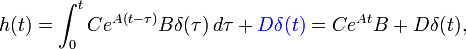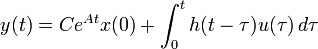# Errata: Impulse response does not include the direct term in the proper manner

(diff) ← Older revision | Latest revision (diff) | Newer revision → (diff)
In equation (5.18) and the subsequent text, the impulse response does not properly account for the direct () term. The corrected text should read:where the second equality follows from the fact that $\delta(t)$ is zero everywhere except the origin and its integral is identically 1. We can now write the convolution equation in terms of the initial condition response and the convolution of the impulse response and the input signal, and the direct term:+ D u(t)